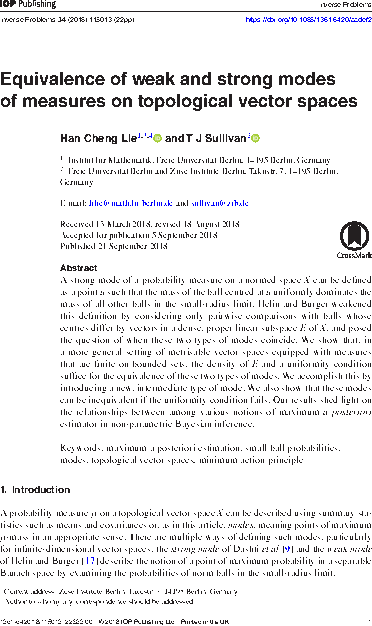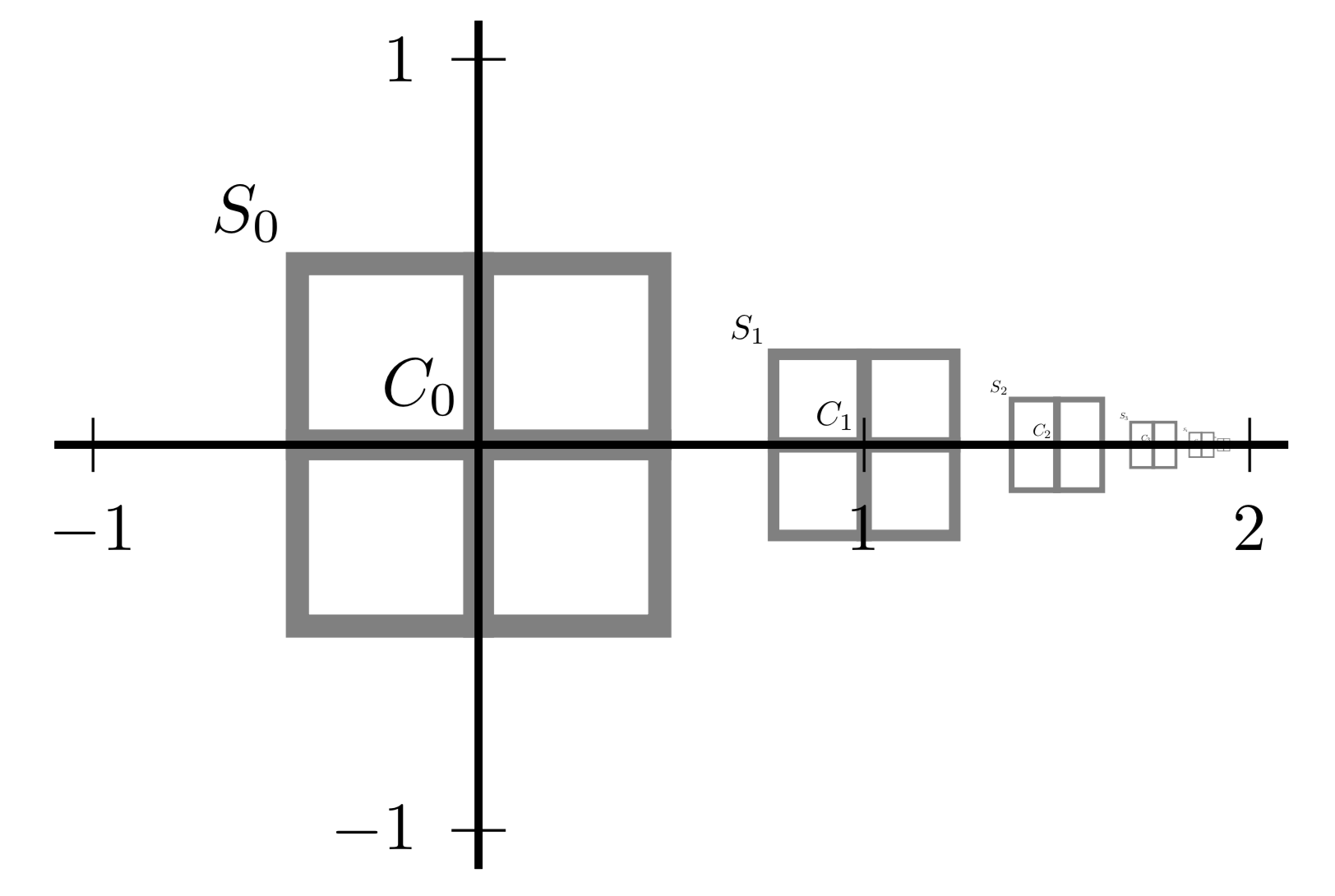# Tim Sullivan

Clear Search

### Birzhan Ayanbayev joins the UQ Group

It is a pleasure to announce that Birzhan Ayanbayev will join the UQ research group as a postdoctoral researcher with effect from 28 February 2020. He will be working on the DFG-funded project “Analysis of maximum a posteriori estimators: Common convergence theories for Bayesian and variational inverse problems”.

Published on Friday 28 February 2020 at 12:00 UTC #group #job #dfg #map-estimators #ayanbayev### Postdoc position: Analysis of MAP estimators

There is still an opening for a full-time two-year postdoctoral research position in the UQ group at the Freie Universität Berlin. This position will be associated to the project “Analysis of maximum a posteriori estimators: Common convergence theories for Bayesian and variational inverse problems” funded by the DFG.

This project aims to advance the state of the art in rigorous mathematical understanding of MAP estimators in infinite-dimensional statistical inverse problems. In particular, the research in this project will connect the “small balls” approach of Dashti et al. (2013) to the calculus of variations and hence properly link the variational and fully Bayesian points of view on inverse problems. This is an exciting opportunity for someone well-versed in the calculus of variations and tools such as Γ-convergence to make an impact on fundamental questions of non-parametric statistics and inverse problems or, vice versa, for someone with a statistical inverse problems background to advance the rigorous state of the art for such methods.

Prospective candidates are encouraged to contact me with informal enquiries. Formal applications are to be sent by post or email, by 23 December 2019, under the heading MAP-Analysis, and should include a cover letter, a scientific CV including list of publications and research statement, and the contact details of two professional references.

Published on Monday 25 November 2019 at 08:00 UTC #group #job #fu-berlin #inverse-problems #dfg #map-estimators### Weak and strong modes in Inverse Problems

The paper “Equivalence of weak and strong modes of measures on topological vector spaces” by Han Cheng Lie and myself has now appeared in Inverse Problems. This paper addresses a natural question in the theory of modes (or maximum a posteriori estimators, in the case of posterior measure for a Bayesian inverse problem) in an infinite-dimensional space $$X$$. Such modes can be defined either strongly (a la Dashti et al. (2013), via a global maximisation) or weakly (a la Helin and Burger (2015), via a dense subspace $$E \subset X$$). The question is, when are strong and weak modes equivalent? The answer turns out to be rather subtle: under reasonable uniformity conditions, the two kinds of modes are indeed equivalent, but finite-dimensional counterexamples exist when the uniformity conditions fail.

H. C. Lie and T. J. Sullivan. “Equivalence of weak and strong modes of measures on topological vector spaces.” Inverse Problems 34(11):115013, 2018. doi:10.1088/1361-6420/aadef2

(See also H. C. Lie and T. J. Sullivan. “Erratum: Equivalence of weak and strong modes of measures on topological vector spaces (2018 Inverse Problems 34 115013).” Inverse Problems 34(12):129601, 2018. doi:10.1088/1361-6420/aae55b )

Abstract. A strong mode of a probability measure on a normed space $$X$$ can be defined as a point $$u \in X$$ such that the mass of the ball centred at $$u$$ uniformly dominates the mass of all other balls in the small-radius limit. Helin and Burger weakened this definition by considering only pairwise comparisons with balls whose centres differ by vectors in a dense, proper linear subspace $$E$$ of $$X$$, and posed the question of when these two types of modes coincide. We show that, in a more general setting of metrisable vector spaces equipped with non-atomic measures that are finite on bounded sets, the density of $$E$$ and a uniformity condition suffice for the equivalence of these two types of modes. We accomplish this by introducing a new, intermediate type of mode. We also show that these modes can be inequivalent if the uniformity condition fails. Our results shed light on the relationships between among various notions of maximum a posteriori estimator in non-parametric Bayesian inference.

Published on Saturday 22 September 2018 at 12:00 UTC #publication #inverse-problems #modes #map-estimators #lie### Weak and strong modes

Han Cheng Lie and I have just uploaded a revised preprint of our paper, “Equivalence of weak and strong modes of measures on topological vector spaces”, to the arXiv. This addresses a natural question in the theory of modes (or maximum a posteriori estimators, in the case of posterior measure for a Bayesian inverse problem) in an infinite-dimensional space $$X$$. Such modes can be defined either strongly (a la Dashti et al. (2013), via a global maximisation) or weakly (a la Helin and Burger (2015), via a dense subspace $$E \subset X$$). The question is, when are strong and weak modes equivalent? The answer turns out to be rather subtle: under reasonable uniformity conditions, the two kinds of modes are indeed equivalent, but finite-dimensional counterexamples exist when the uniformity conditions fail.

Abstract. A strong mode of a probability measure on a normed space $$X$$ can be defined as a point $$u \in X$$ such that the mass of the ball centred at $$u$$ uniformly dominates the mass of all other balls in the small-radius limit. Helin and Burger weakened this definition by considering only pairwise comparisons with balls whose centres differ by vectors in a dense, proper linear subspace $$E$$ of $$X$$, and posed the question of when these two types of modes coincide. We show that, in a more general setting of metrisable vector spaces equipped with non-atomic measures that are finite on bounded sets, the density of $$E$$ and a uniformity condition suffice for the equivalence of these two types of modes. We accomplish this by introducing a new, intermediate type of mode. We also show that these modes can be inequivalent if the uniformity condition fails. Our results shed light on the relationships between among various notions of maximum a posteriori estimator in non-parametric Bayesian inference.

Published on Monday 9 July 2018 at 08:00 UTC #preprint #inverse-problems #modes #map-estimators #lie